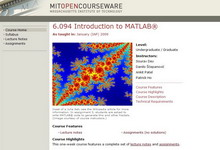###### >>查看 资源 属性
 资源名称 资源来源6.094 Introduction to MATLAB？ January(IAP) 2009 麻省理工学院 工学学科 Sourav Dey,Danilo ？？epanovi？,Ankit Patel,Patrick Ho 本科生,硕士研究生 matlab   simulink   matlab programming   variables   plotting   scripts   functions   flow control   linear algebra   polynomials   optimization   differential equations   ode   probability   statistics   data structures   images   animation   debugging   symbolic math   toolboxes   scope   function block   nervous system This course provides an aggressively gentle introduction to MATLAB？. It is designed to give students fluency in MATLAB, including popular toolboxes. The course consists of interactive lectures with a computer running MATLAB for each student. Problem-based MATLAB assignments are given which require significant time on MATLAB. 浏览次数：5169, 下载次数：24 2010-04-21